# RD Sharma Solutions for Class 8 Maths Chapter 1 - Rational Numbers Exercise 1.1

Students can refer and download RD Sharma Solutions for Class 8 Maths Exercise 1.1 Chapter 1, Rational Numbers from the links provided below.

Our experts have solved the RD Sharma Solutions to ensure that the students are thorough with their basic concepts and help clear their doubts. Exercise 1.1 is based on the basic concepts of rational numbers with the addition of same and different denominators. Students can download the RD Sharma Class 8 Maths Chapter 1 and start practising offline.

## Download PDF of RD Sharma Solutions for Class 8 Maths Exercise 1.1 Chapter 1 Rational Numbers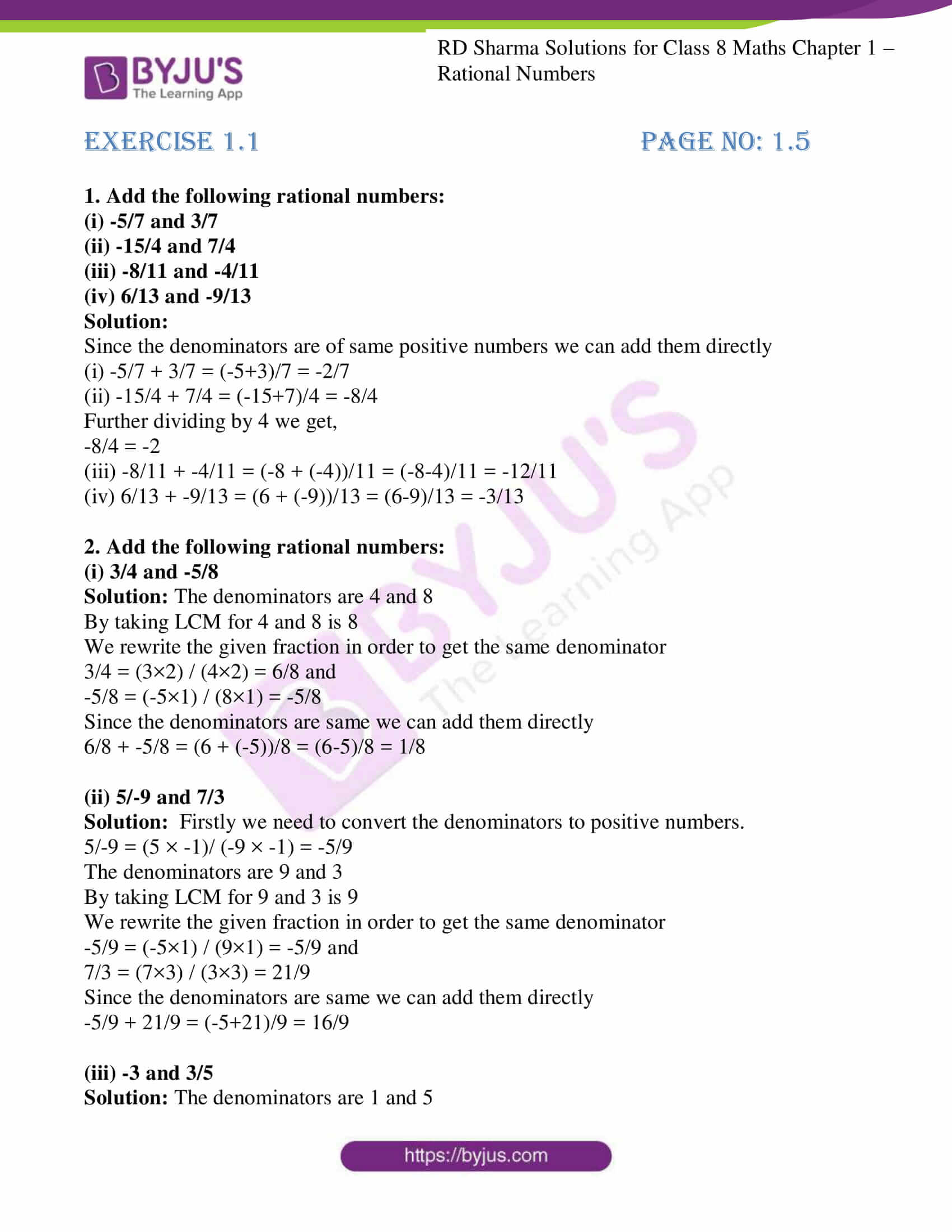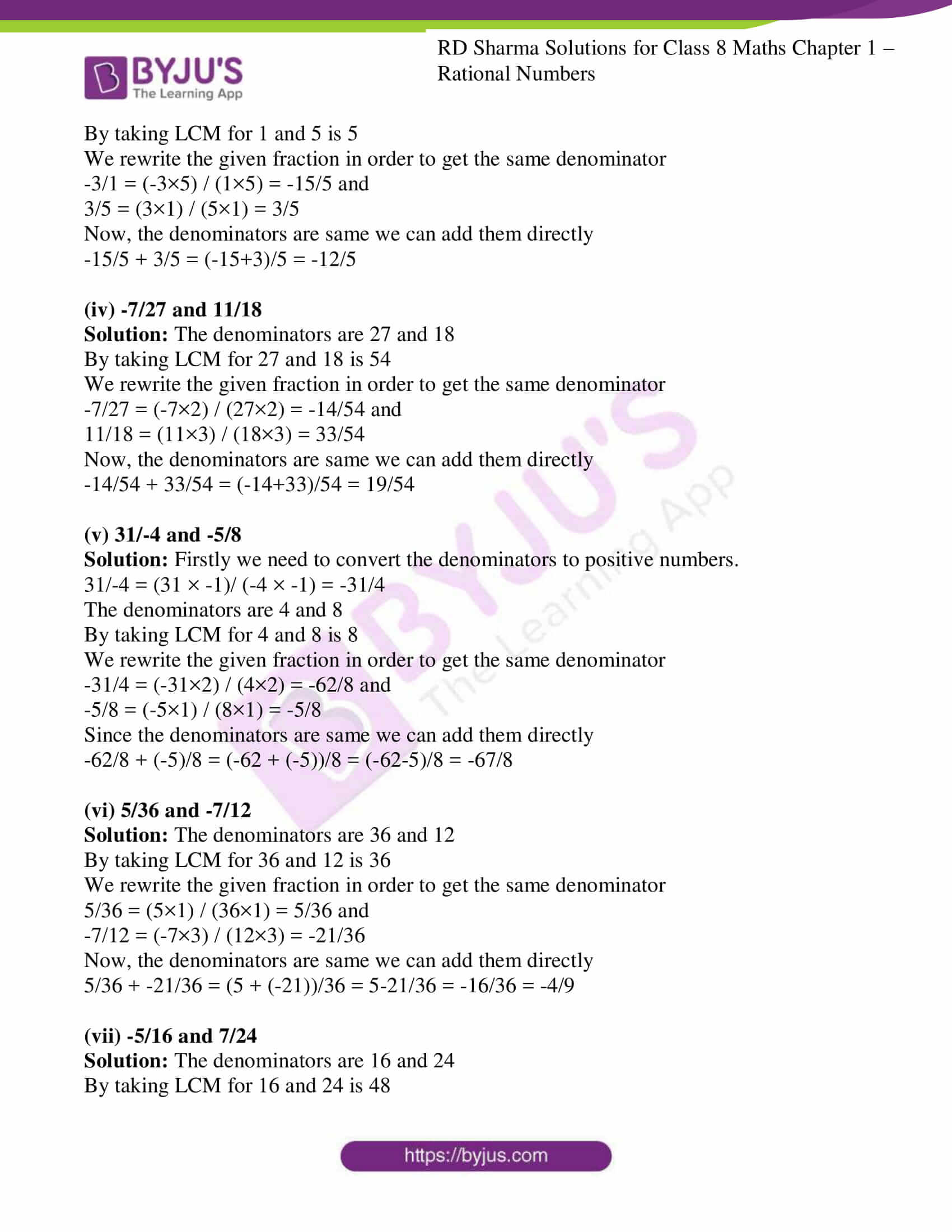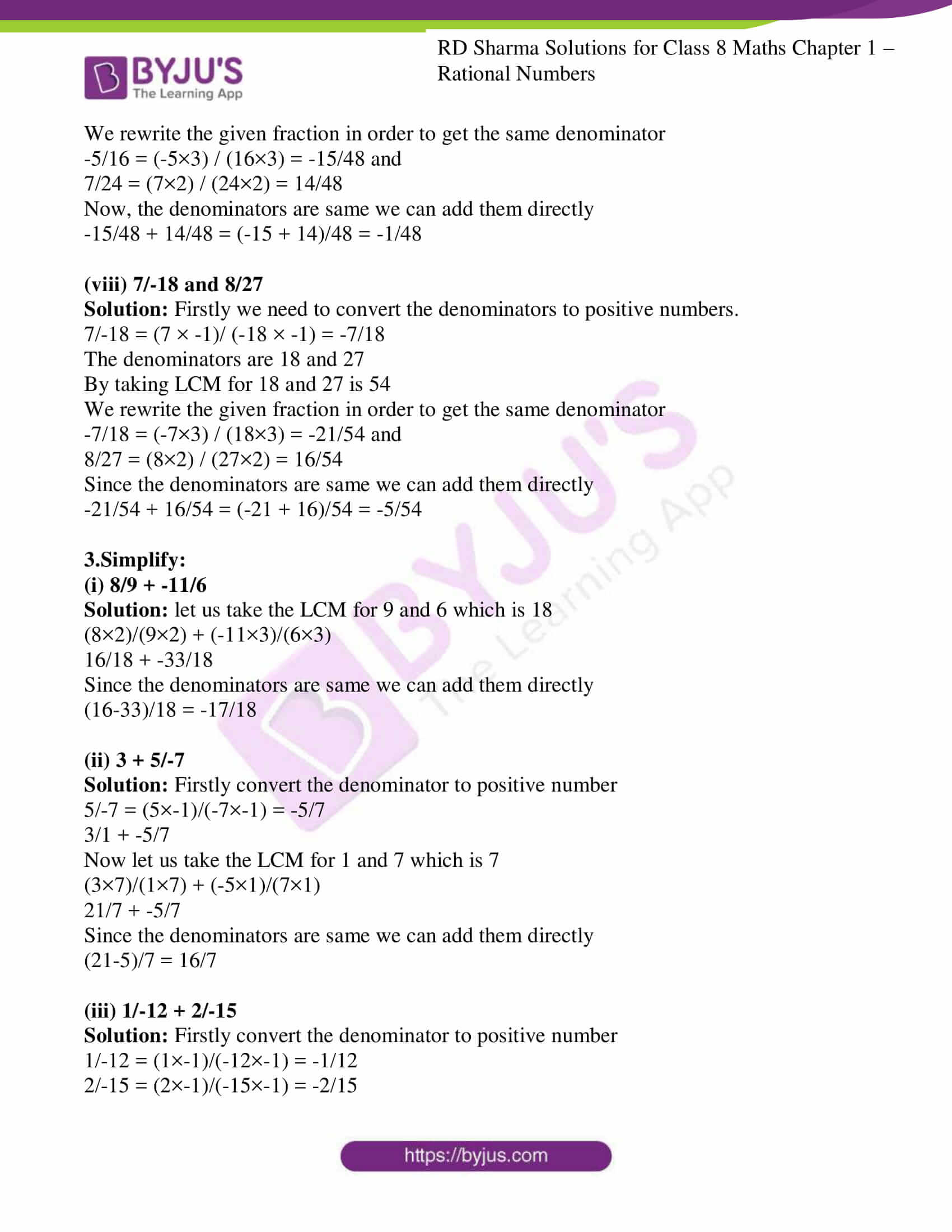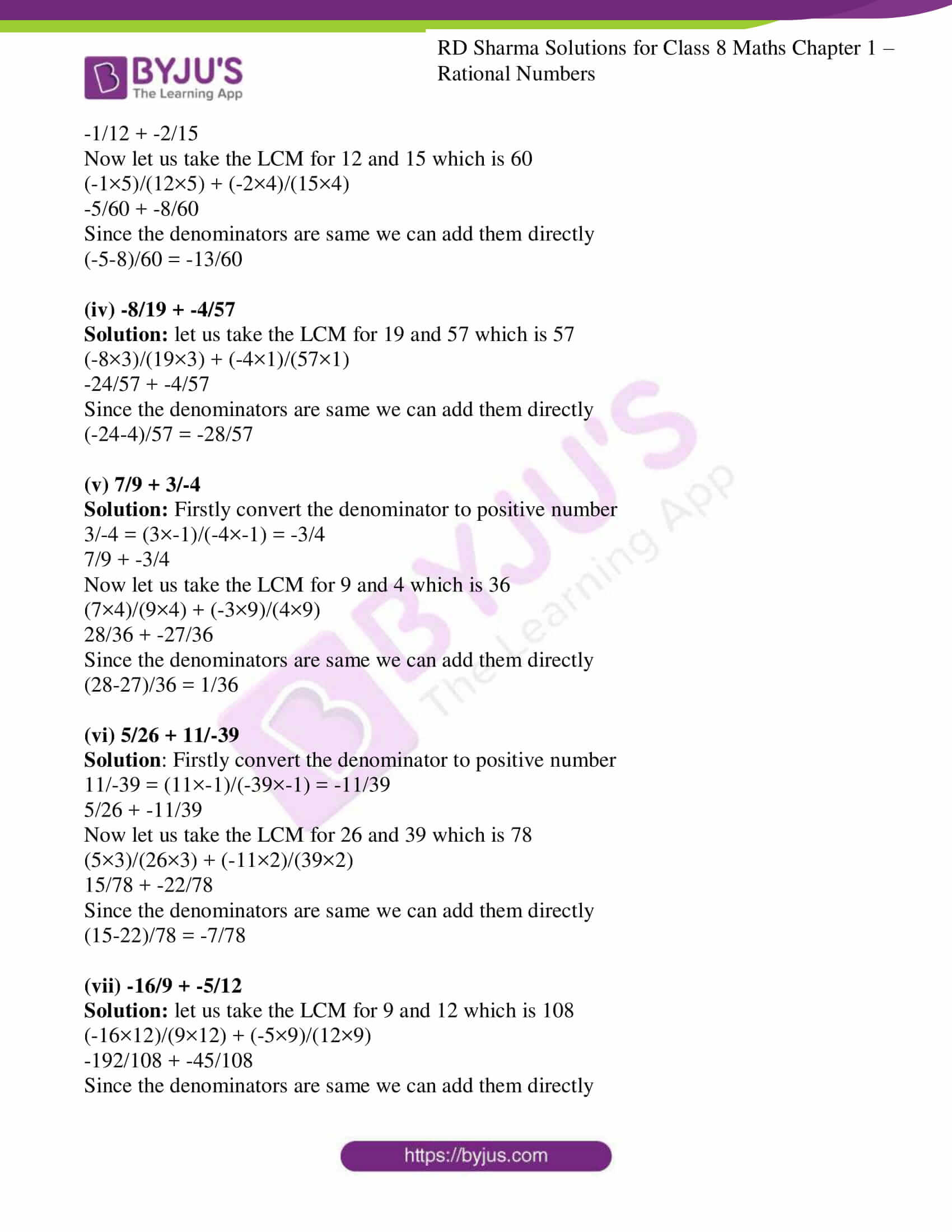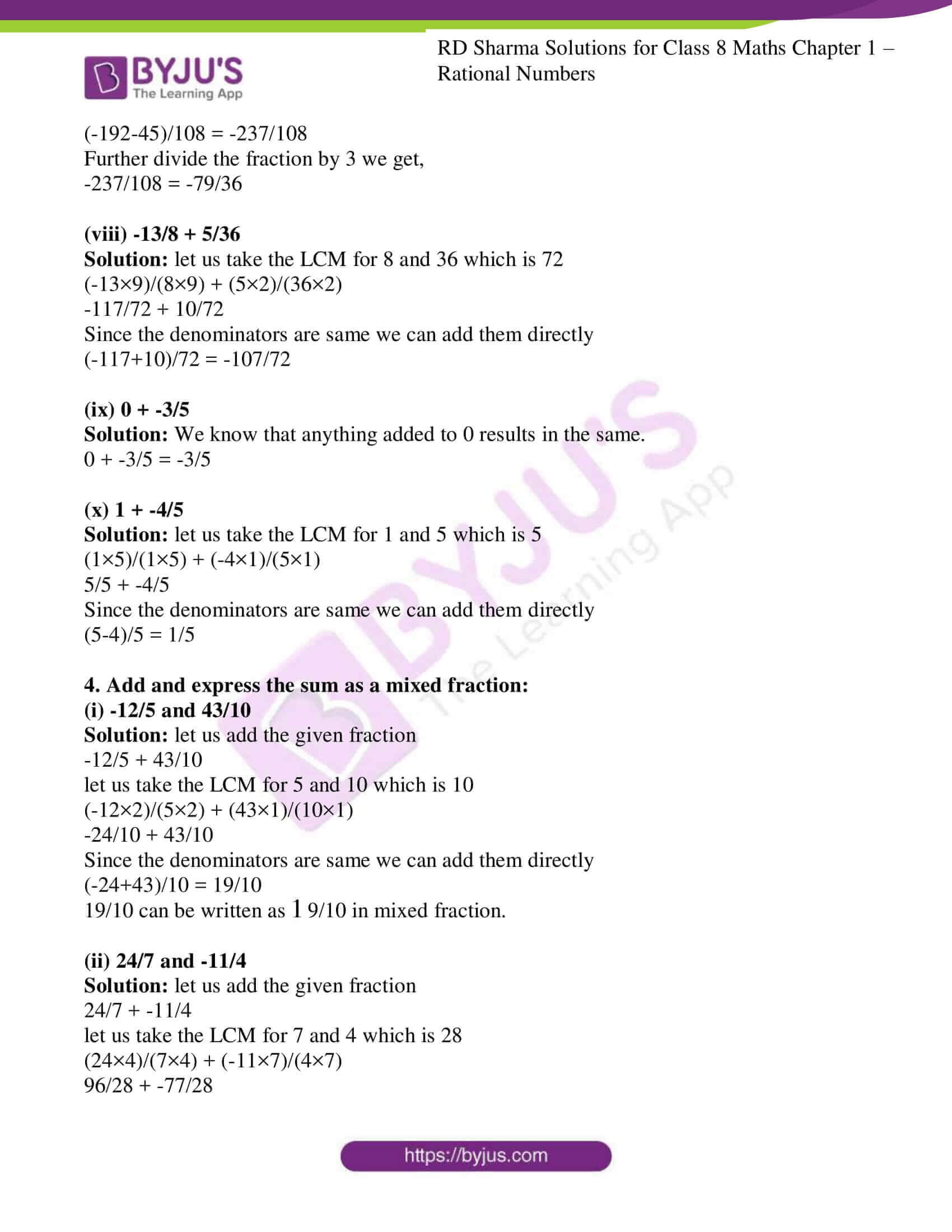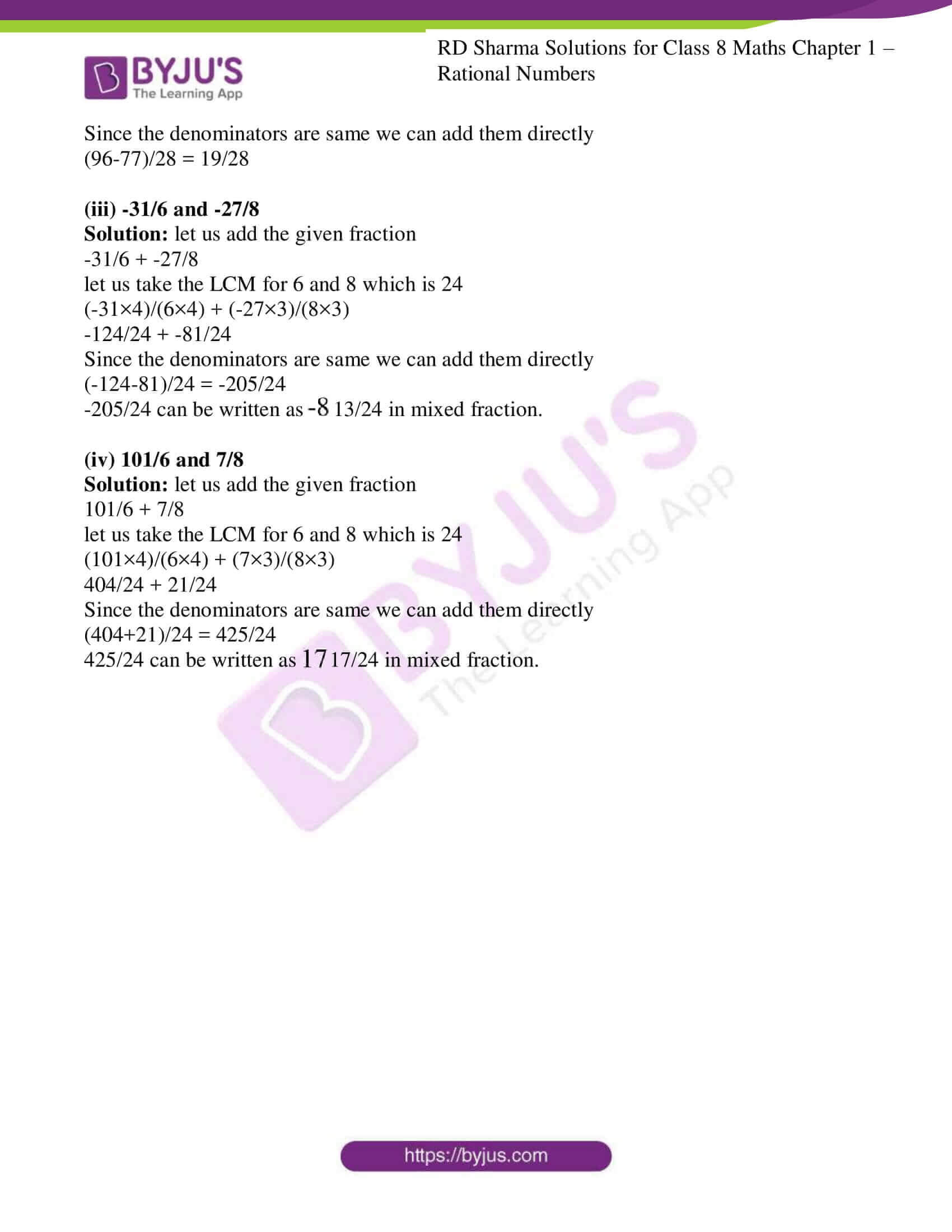### Access Answers to RD Sharma Solutions for Class 8 Maths Exercise 1.1 Chapter 1 Rational Numbers

1. Add the following rational numbers:

(i) -5/7 and 3/7

(ii) -15/4 and 7/4

(iii) -8/11 and -4/11

(iv) 6/13 and -9/13

Solution:

Since the denominators are of same positive numbers we can add them directly

(i) -5/7 + 3/7 = (-5+3)/7 = -2/7

(ii) -15/4 + 7/4 = (-15+7)/4 = -8/4

Further dividing by 4 we get,

-8/4 = -2

(iii) -8/11 + -4/11 = (-8 + (-4))/11 = (-8-4)/11 = -12/11

(iv) 6/13 + -9/13 = (6 + (-9))/13 = (6-9)/13 = -3/13

2. Add the following rational numbers:

(i) 3/4 and -5/8

Solution: The denominators are 4 and 8

By taking LCM for 4 and 8 is 8

We rewrite the given fraction in order to get the same denominator

3/4 = (3×2) / (4×2) = 6/8 and

-5/8 = (-5×1) / (8×1) = -5/8

Since the denominators are same we can add them directly

6/8 + -5/8 = (6 + (-5))/8 = (6-5)/8 = 1/8

(ii) 5/-9 and 7/3

Solution: Firstly we need to convert the denominators to positive numbers.

5/-9 = (5 × -1)/ (-9 × -1) = -5/9

The denominators are 9 and 3

By taking LCM for 9 and 3 is 9

We rewrite the given fraction in order to get the same denominator

-5/9 = (-5×1) / (9×1) = -5/9 and

7/3 = (7×3) / (3×3) = 21/9

Since the denominators are same we can add them directly

-5/9 + 21/9 = (-5+21)/9 = 16/9

(iii) -3 and 3/5

Solution: The denominators are 1 and 5

By taking LCM for 1 and 5 is 5

We rewrite the given fraction in order to get the same denominator

-3/1 = (-3×5) / (1×5) = -15/5 and

3/5 = (3×1) / (5×1) = 3/5

Now, the denominators are same we can add them directly

-15/5 + 3/5 = (-15+3)/5 = -12/5

(iv) -7/27 and 11/18

Solution: The denominators are 27 and 18

By taking LCM for 27 and 18 is 54

We rewrite the given fraction in order to get the same denominator

-7/27 = (-7×2) / (27×2) = -14/54 and

11/18 = (11×3) / (18×3) = 33/54

Now, the denominators are same we can add them directly

-14/54 + 33/54 = (-14+33)/54 = 19/54

(v) 31/-4 and -5/8

Solution: Firstly we need to convert the denominators to positive numbers.

31/-4 = (31 × -1)/ (-4 × -1) = -31/4

The denominators are 4 and 8

By taking LCM for 4 and 8 is 8

We rewrite the given fraction in order to get the same denominator

-31/4 = (-31×2) / (4×2) = -62/8 and

-5/8 = (-5×1) / (8×1) = -5/8

Since the denominators are same we can add them directly

-62/8 + (-5)/8 = (-62 + (-5))/8 = (-62-5)/8 = -67/8

(vi) 5/36 and -7/12

Solution: The denominators are 36 and 12

By taking LCM for 36 and 12 is 36

We rewrite the given fraction in order to get the same denominator

5/36 = (5×1) / (36×1) = 5/36 and

-7/12 = (-7×3) / (12×3) = -21/36

Now, the denominators are same we can add them directly

5/36 + -21/36 = (5 + (-21))/36 = 5-21/36 = -16/36 = -4/9

(vii) -5/16 and 7/24

Solution: The denominators are 16 and 24

By taking LCM for 16 and 24 is 48

We rewrite the given fraction in order to get the same denominator

-5/16 = (-5×3) / (16×3) = -15/48 and

7/24 = (7×2) / (24×2) = 14/48

Now, the denominators are same we can add them directly

-15/48 + 14/48 = (-15 + 14)/48 = -1/48

(viii) 7/-18 and 8/27

Solution: Firstly we need to convert the denominators to positive numbers.

7/-18 = (7 × -1)/ (-18 × -1) = -7/18

The denominators are 18 and 27

By taking LCM for 18 and 27 is 54

We rewrite the given fraction in order to get the same denominator

-7/18 = (-7×3) / (18×3) = -21/54 and

8/27 = (8×2) / (27×2) = 16/54

Since the denominators are same we can add them directly

-21/54 + 16/54 = (-21 + 16)/54 = -5/54

3.Simplify:

(i) 8/9 + -11/6

Solution: let us take the LCM for 9 and 6 which is 18

(8×2)/(9×2) + (-11×3)/(6×3)

16/18 + -33/18

Since the denominators are same we can add them directly

(16-33)/18 = -17/18

(ii) 3 + 5/-7

Solution: Firstly convert the denominator to positive number

5/-7 = (5×-1)/(-7×-1) = -5/7

3/1 + -5/7

Now let us take the LCM for 1 and 7 which is 7

(3×7)/(1×7) + (-5×1)/(7×1)

21/7 + -5/7

Since the denominators are same we can add them directly

(21-5)/7 = 16/7

(iii) 1/-12 + 2/-15

Solution: Firstly convert the denominator to positive number

1/-12 = (1×-1)/(-12×-1) = -1/12

2/-15 = (2×-1)/(-15×-1) = -2/15

-1/12 + -2/15

Now let us take the LCM for 12 and 15 which is 60

(-1×5)/(12×5) + (-2×4)/(15×4)

-5/60 + -8/60

Since the denominators are same we can add them directly

(-5-8)/60 = -13/60

(iv) -8/19 + -4/57

Solution: let us take the LCM for 19 and 57 which is 57

(-8×3)/(19×3) + (-4×1)/(57×1)

-24/57 + -4/57

Since the denominators are same we can add them directly

(-24-4)/57 = -28/57

(v) 7/9 + 3/-4

Solution: Firstly convert the denominator to positive number

3/-4 = (3×-1)/(-4×-1) = -3/4

7/9 + -3/4

Now let us take the LCM for 9 and 4 which is 36

(7×4)/(9×4) + (-3×9)/(4×9)

28/36 + -27/36

Since the denominators are same we can add them directly

(28-27)/36 = 1/36

(vi) 5/26 + 11/-39

Solution: Firstly convert the denominator to positive number

11/-39 = (11×-1)/(-39×-1) = -11/39

5/26 + -11/39

Now let us take the LCM for 26 and 39 which is 78

(5×3)/(26×3) + (-11×2)/(39×2)

15/78 + -22/78

Since the denominators are same we can add them directly

(15-22)/78 = -7/78

(vii) -16/9 + -5/12

Solution: let us take the LCM for 9 and 12 which is 108

(-16×12)/(9×12) + (-5×9)/(12×9)

-192/108 + -45/108

Since the denominators are same we can add them directly

(-192-45)/108 = -237/108

Further divide the fraction by 3 we get,

-237/108 = -79/36

(viii) -13/8 + 5/36

Solution: let us take the LCM for 8 and 36 which is 72

(-13×9)/(8×9) + (5×2)/(36×2)

-117/72 + 10/72

Since the denominators are same we can add them directly

(-117+10)/72 = -107/72

(ix) 0 + -3/5

Solution: We know that anything added to 0 results in the same.

0 + -3/5 = -3/5

(x) 1 + -4/5

Solution: let us take the LCM for 1 and 5 which is 5

(1×5)/(1×5) + (-4×1)/(5×1)

5/5 + -4/5

Since the denominators are same we can add them directly

(5-4)/5 = 1/5

4. Add and express the sum as a mixed fraction:

(i) -12/5 and 43/10

Solution: let us add the given fraction

-12/5 + 43/10

let us take the LCM for 5 and 10 which is 10

(-12×2)/(5×2) + (43×1)/(10×1)

-24/10 + 43/10

Since the denominators are same we can add them directly

(-24+43)/10 = 19/10

19/10 can be written as

$$\begin{array}{l}1\frac{9}{10}\end{array}$$
in mixed fraction.

(ii) 24/7 and -11/4

Solution: let us add the given fraction

let us take the LCM for 7 and 4 which is 28

(24×4)/(7×4) + (-11×7)/(4×7)

96/28 + -77/28

Since the denominators are same we can add them directly

(96-77)/28 = 19/28

(iii) -31/6 and -27/8

Solution: let us add the given fraction

-31/6 + -27/8

let us take the LCM for 6 and 8 which is 24

(-31×4)/(6×4) + (-27×3)/(8×3)

-124/24 + -81/24

Since the denominators are same we can add them directly

(-124-81)/24 = -205/24

-205/24 can be written as

$$\begin{array}{l}-8\frac{13}{24}\end{array}$$
in mixed fraction.

(iv) 101/6 and 7/8

Solution: let us add the given fraction

101/6 + 7/8

let us take the LCM for 6 and 8 which is 24

(101×4)/(6×4) + (7×3)/(8×3)

404/24 + 21/24

Since the denominators are same we can add them directly

(404+21)/24 = 425/24

425/24 can be written as

$$\begin{array}{l}17\frac{17}{24}\end{array}$$
in mixed fraction.

## RD Sharma Solutions for Class 8 Maths Exercise 1.1 Chapter 1 Rational Numbers

Exercise 1.1 of RD Sharma Class 8 Chapter 1, Rational Numbers contains the basic concepts related to Rational Numbers. This exercise mainly deals with the basic properties of Rational Numbers. Some of them include

• Equivalent Rational Numbers
• Positive and negative Rational Numbers
• Comparison of Rational Numbers

The RD Sharma Solutions can help the students practice diligently while learning the fundamentals as it provides all the answers to the questions from the RD Sharma textbook. Practising as many times as possible helps in scoring high marks.